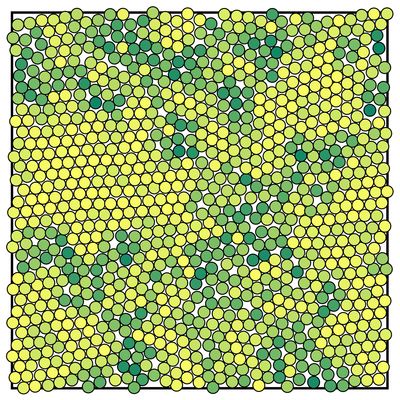## Structural order parameters (the easy way)

A simple means of computing local structural order in a two-dimensional atomistic system is by use of bond order parameters such as

$$\psi_l^j=\frac{1}{n_j}\sum_k \mathrm{exp}\left(i\ell\theta_{jk}\right),$$

where $$n_j$$ is the number of neighbours within the first atom shell of particle $$j$$ and $$\theta_{jk}$$ is the angle between the vector connecting particles $$j$$ and $$k$$ and the horizontal axis. The parameter $$\ell$$ specifies the type of symmetry in which we are interested, e.g. $$\ell=6$$ for six-fold symmetry (hexatic order), as illustrated in the image below (particles colored yellow have a high degree of hexatic order).Computing such an order parameter typically requires calculation of the angles between all of the nearest neighbour particle pairs. One must first take an arctangent (or similar) to compute the angle and then use sines and cosines to evaluate the exponential factor. Such a procedure is rather computationally expensive, particularly when a large number of statistics are required and one must consider all particles in the system at multiple time steps. However, there is a useful trick that lets us evaluate the expression without having to compute a single pesky sine or cosine.

$$e^{i(x)}=\mathrm{cos}\left(x\right) + i \mathrm{sin}\left(x\right).$$

Combining with the exponential law for integer powers leads us to de Moivre's formula

\begin{eqnarray*} \left(e^{ix}\right)^n&=&e^{inx}\\ e^{i(nx)}&=&\mathrm{cos}\left(nx\right) + i \mathrm{sin}\left(nx\right)\\ \mathrm{cos}\left(nx\right) + i \mathrm{sin}\left(nx\right)&=&\left[\mathrm{cos}(x) + i\mathrm{sin}(x)\right]^n. \end{eqnarray*}

This is nothing more than a binomial expansion. Letting $$x=\theta_{jk}$$ and considering a hexatic order parameter gives

$$e^{i6x}\equiv\mathrm{cos}\left(6x\right) + i \mathrm{sin}\left(6x\right)\equiv\left[\mathrm{cos}(x) + i\mathrm{sin}(x)\right]^6.$$

The coefficients for a power six binomial expansion are as follows,

$$1,\ 6,\ 15,\ 20,\ 15,\ 6,\ 1.$$

If we let $$c=\mathrm{cos}\left(x\right)$$ and $$s=\mathrm{sin}\left(x\right)$$ then evaluating the real and imaginary components gives

\begin{eqnarray*} \Re \left\{e^{i6x}\right\} &=& c^6 - 15c^4s^2 + 15c^2s^4 - s^6\\ \Im \left\{e^{i6x}\right\} &=& 6c^5s - 20c^3s^3 + 6cs^5 \end{eqnarray*}

In any molecular dynamics integrator one must compute particle separations in order to evaluate forces due to the pair potential. For example, in two dimensions one would measure the magnitude of the separation vector $$\mathbf{r}$$ as well as the $$x$$ and $$y$$ components, $$\Delta x$$ and $$\Delta y$$ respectively. These values can be used to compute the sine and cosine of the angle between the pair vector and horizontal which in turn can be used in the above expressions to compute the desired structural order parameter, i.e.

\begin{eqnarray*} \mathrm{cos}\left(\theta_{jk}\right)&=&\Delta x_{jk}/|\mathbf{r}_{jk}|\\ \mathrm{sin}\left(\theta_{jk}\right)&=&\Delta y_{jk}/|\mathbf{r}_{jk}| \end{eqnarray*}

This method allows structural order parameters to be computed on-the-fly within any molecular dynamics integrator with little additional computational burden.